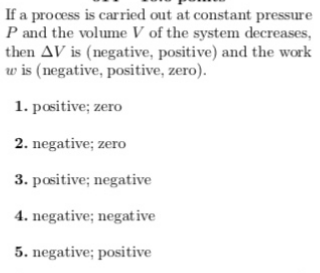# If a process is carried out at constant pressure P and the volume V of the system decreases, then ΔV is (negative, positive) and the work w is (negative, positive, zero). 1. positive; zero 2. negative; zero 3. positive; negative 4. negative; negative 5. negative; positive